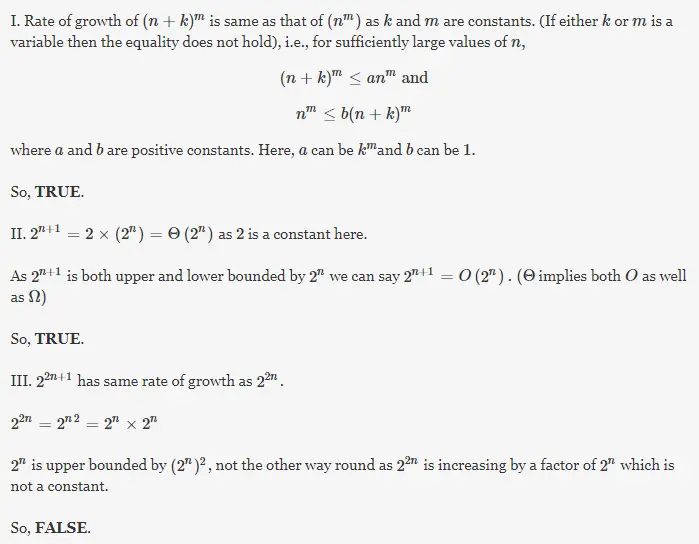# Gate CS-2003 Question Paper With Solutions

Q. 1 Consider the following three claims

```1.(n + k)m = Θ(nm), where k and m are constants

2. 2n + 1 = O(2n)

3. 22n + 1 = O(2n)```

Which of these claims are correct?

(A) 1 and 2

(B) 1 and 3

(C) 2 and 3

(D) 1, 2 and 3